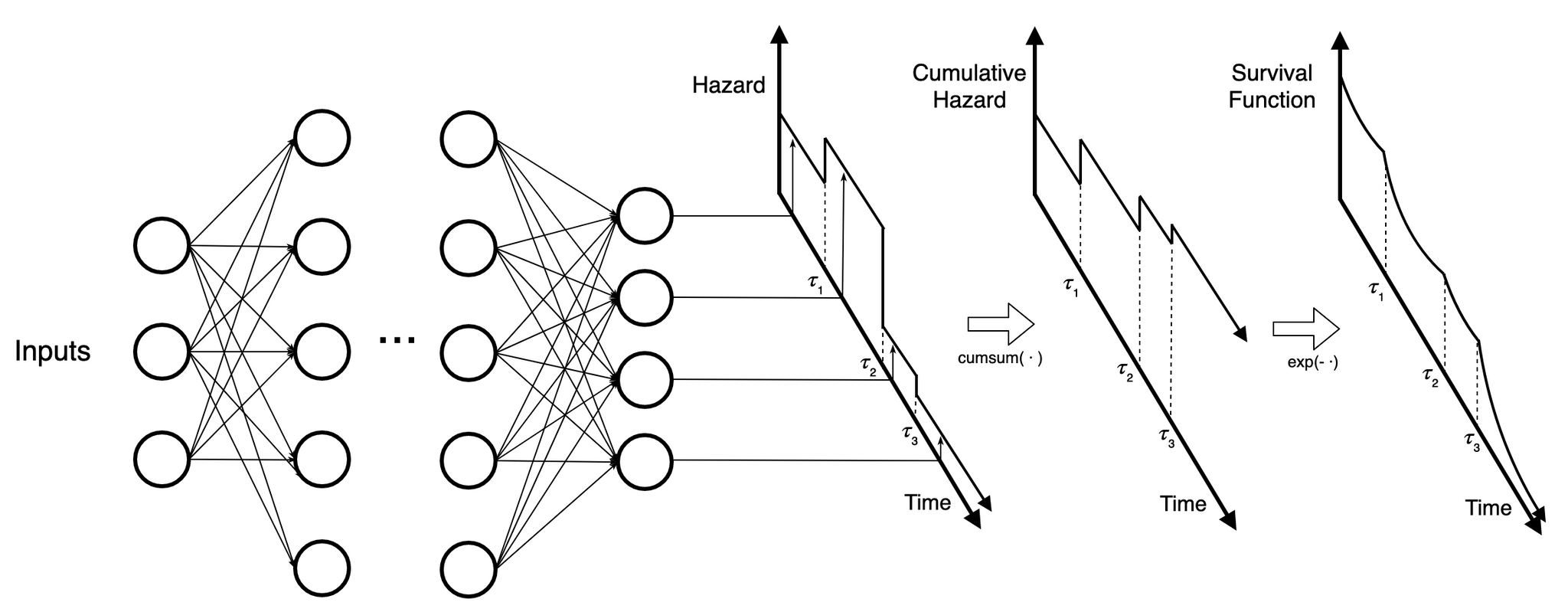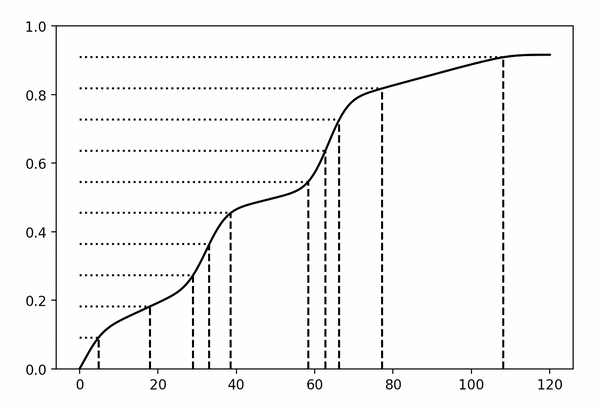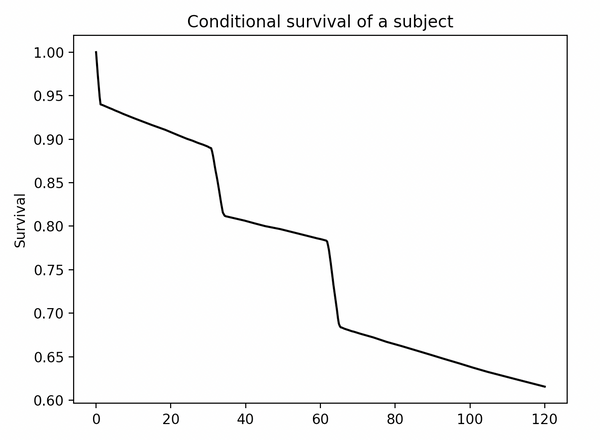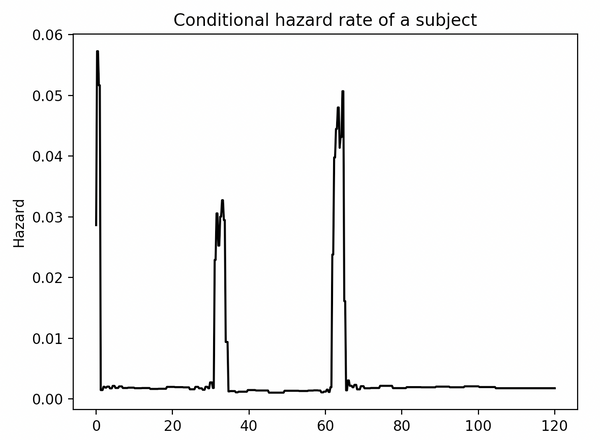# Non-parametric survival function prediction

Posted by Cameron Davidson-Pilon on

As I was developing lifelines, I kept having a feeling that I was gradually moving the library towards prediction tasks. lifelines is great for regression models and fitting survival distributions, but as I was adding more and more flexible parametric models, I realized that I really wanted a model that would predict the survival function — and I didn't care how. This led me to the idea to use a neural net with $$n$$ outputs, one output for each parameter in the cumulative hazard, $$H(t)$$. lifelines has implemented the linear version of this, where each parameter in the cumulative hazard is a linear function of the variables:

Linear:

$$H(t\;|\;x) = f(a, b, c) \\a = a(x \cdot \beta_1^T) \\b = b(x \cdot \beta_2^T) \\c = c(x \cdot \mathbf{\beta}_3^T)$$

Neural Net (NN):

$$H(t\;|\;x) = f(a, b, c) \\a = \text{NN}(x)_1 \\b = \text{NN}(x)_2 \\c = \text{NN}(x)_3$$

If my NN has no hidden layer, it's equivalent to the linear case. Great, so using a NN and a function $$f$$ provides us a good way to predict survival functions. Can we do better? Well, we are stuck with a particular $$f$$, which may or may not be flexible enough to capture all the nuances of possible survival. For example, SaaS churn has very unique survival behavior that a traditional parametric model couldn't capture well.

Thinking more about that blog post linked above, I realized that I could use a piecewise approach and split my timescale into partitions as fine as I want, and then predict a constant hazard in each interval. Graphically, something like this:Each output predicts the hazard in a time interval. This piecewise constant hazard can form the cumulative hazard, and then the survival function.

As the NN increases in depth and complexity, each output benefits from the information in the other time periods. For example, if the model thinks that a certain generated feature is really important, it's exposed for all outputs to use.

What about those taus — which I call breakpoints — how are they determined? There are a few options. The first option is the manually specify them. This is okay if we have apriori knowledge of important times, but often we don't. A second option is to expand the neural net to return breakpoints as well. Unfortunately I haven't gotten this model to converge well. The final option considered is to use the dataset to choose breakpoints. In the Kaplan Meier model, the time points of change in the survival function are equal to the unique event times. More generally, the density of the changes in survival is proportional to the density of observed event times. Using this, we can programmatically choose good breakpoints by first creating a density estimate of observed event times, and spacing out breakpoints in proportion to high density. That is, we solve $$p=F_T(t)$$ for $$t$$ at evenly spaced $$p$$ values. Below is an example CDF and how breakpoints are determined:The number of breakpoints is more arbitrary, and can be manually set or heuristically set (like proportional to the square root the number of observed event times).

### Implementation in Python

So, to recap: we choose breakpoints in proportion to density of event times (which is where the most information is), and use a neural net to determine a subject's hazard in that interval. This is exactly what my new Python library, implements. At the moment, I am building upon the computational library Jax. lifelike's API is similar to Keras, and users familiar with Keras (and Jax) could jump in immediately. The library is also quite opinionated, and based on my own philosophy on survival analysis. For example:

1. The predicted survival function is returned, and no effort is made to compute a point estimate. This aligns with my belief that a distribution has enormous advantages (and on the other side of that coin: point estimates are overrated). Users can compute their own points estimates if they wish

2. Fitting is done against the log-likelihood loss. The survival analysis log-likelihood contains all the information around censoring and observed event times. Is it interpretable? Not really, but most losses are not anyways. The goal is to minimize loss, and I believe the log-likelihood is a terrific loss.

Let's see some inference done with lifelike. The model was trained on an artificial SaaS churn dataset. No prior knowledge about the breakpoints were provided. Below we see the predicted survival function and predicted hazard of a subject.The model was correctly able to find the periods of high SaaS customer churn, and predict the subject's survival function.

### Conclusion

This NN piecewise-constant model isn't new, and has been published recently in literature before. However, almost all the literature has a very shallow network, and is fit using BFGS. lifelike is the first implementation in a modern computational graph framework, and leaning on Jax means we get autodiff, JIT and GPUs for free. We can scale this model is any dataset size or network we wish (limited only by Jax). For example, my upcoming research could be using images from spectrometers as an input to NNs — lifelike theoretically can handle that.

I hope this blog article inspired you or gave you some new ideas. lifelike is very experimental, and I'm still iterating on APIs and functionality, so use at your own risk. Stay tuned for more updates!